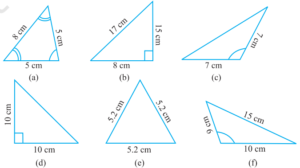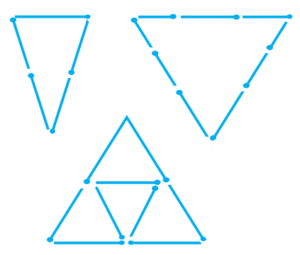# Ncert Class 6 Math Understanding Elementary Shapes Exercise 5.6

Understanding Elementary Shapes Class 6 Ex. 5.6

New Ncert Class 6 Math Free Solution.

Exercise 5.6

Question 1 :- Name the types of following triangles:
(а) Triangle with the lengths of sides 7 cm, 8 cm and 9 cm.
(b) ∆ABC;- with AB = 8.7 cm, AC = 7 cm and BC = 6 cm.
(c) ∆PQR; such that PQ = QR = PR = 5 cm.
(d) ∆DEF; with m∠D = 90°
(e) ∆XYZ;- with m∠Y = 90° and XY = YZ.
(f) ∆LMN;- with m∠L = 30° m∠M = 70° and m∠N = 80°.

Solution 1:-
(a) Since, all sides of the given triangle are different.
Hence, it is a Scalene triangle.
(b) Here AB ≠ AC ≠ BC.
Hence, ∆ABC is Scalene triangle.
(c) Since all sides are equal.
Hence, it is an equilateral triangle.
(d)  it is a right angled triangle.
(e)  it is a right angled triangle.
(f)   it is an acute angled triangle.

Question 2 :- Match the following :-

Measure of triangle                                                    Type of triangle

(i) 3 sides of equal length                                            (a) Scalene
(ii) 2 sides of equal length                                           (b) Isosceles right angled
(iii) All sides are of different length                            (c) Obtuse angled
(iv) 3 acute angles                                                         (d) Right angled
(v) 1 right angle                                                             (e) Equilateral
(vi) 1 obtuse angle                                                         (f) Acute angled
(vii) 1 right angle with two sides of equal length       (g) Isosceles

Solution 2:-
(i) ↔ (e)
(ii) ↔ (g)
(iii) ↔ (a)
(iv) ↔ (f)
(v) ↔ (d)
(vi) ↔ (c)
(vii) ↔ (b)

Question 3 :- Name-each of the following triangles in two different ways: (You may judge the nature of the angle by the observation) :Solution 3:-
(a) (i) Acute angled triangle
(ii) Isosceles triangle

(b) (i) Right angled triangle
(ii) Scalene triangle

(c) (i) Obtuse angled triangle
(ii) Isosceles triangle

(d) (i) Right angled triangle
(ii) Isosceles triangle

(e) (i) Acute angled triangle
(ii) Equilateral triangle

(f) (i) Obtuse angled triangle
(ii) Scalene triangle.

Question 4 :- Try to construct triangles using matchsticks. Some are shown here. Can you make a triangle with(a) 3 matchsticks?
(b) 4 matchsticks?
(c) 5 matchsticks?
(d) 6 matchsticks?

(Remember you have to use the all the available matchsticks in each case)
Name the type-of-triangle in each-case.
If you cannot make a triangle,you can give of reasons for it.

Solution 4:-
(a)Yes, three matchsticks can be used to create an equilateral triangle.

(b) No, four matchsticks won’t be able to form a triangle.

(c) Yes, five matchsticks can be used to create an isosceles triangle.

(d) Yes, with six matchsticks, we can create an equilateral triangle.